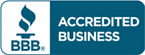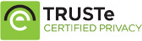Sale!

# Test bank for Business Statistics Communicating with Numbers by Sanjiv Jaggia

\$57.00 \$30.00

Business Statistics Communicating with Numbers by Sanjiv Jaggia Test bank

Full chapters are included

You want Solution Manual?Categories: ,

## Description

Test bank for Business Statistics Communicating with Numbers by Sanjiv Jaggia

Table of content

Chapter 1 Statistics and Data
Chapter 2 Tabular and Graphical Methods
Chapter 3 Numerical Descriptive Measures
Chapter 4 Basic Probability Concepts
Chapter 5 Discrete Probability Distributions
Chapter 6 Continuous Probability Distributions
Chapter 7 Sampling and Sampling Distributions
Chapter 8 Estimation
Chapter 9 Hypothesis Testing
Chapter 10 Statistical Inference Concerning Two Populations
Chapter 11 Statistical Inference Concerning Variance
Chapter 12 Chi-Square Tests
Chapter 13 Analysis of Variance
Chapter 14 Regression Analysis
Chapter 15 Inference with Regression Models
Chapter 16 Regression Models for Nonlinear Relationships
Chapter 17 Regression Models with Dummy Variables
Chapter 18 Time Series and Forecasting
Chapter 19 Returns, Index Numbers and Inflation
Chapter 20 Nonparametric Tests
Appendix A Statistical Tables
Appendix B Solutions to Even-Numbered Exercises
Appendix C Glossary